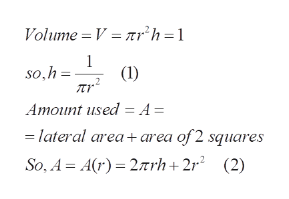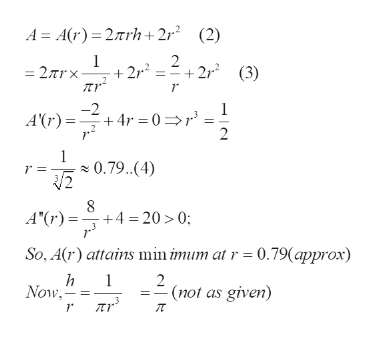Question
34 views

A cylindrical metal can is to be made to hold 1 L of oil. Find the dimensions that will minimize the cost of the metal to manufacture the can. This condition will apply throughout the project. (1 Liter = 1000 cm3)

The material for cans is cut from sheets of metal. The cylindrical sides are formed by bending rectangles. These rectangles are cut from the sheet with little or no waste.

But if the top and bottom discs are cut from squares of side 2(see the figure on the left), this leaves considerable waste material, which may be recycled but has little or no value to the can makers. If this is the case, show that the amount of material used is minimized when (h/r)=(8/pi)= 2.55

Note: Start with the formula for the material required for one can- the lateral surface area + the area of 2 squares.

Part 1 – Write the formula for the value to be minimized and find the critical numbers.

Part 2 – Use the First or Second Derivative test to verify that a critical number will give us the minimum value.

check_circle

star
star
star
star
star
1 Rating
Step 1

To analyze the amount of material to be minimized under the given conditions

Step 2

Formulae to be usedhelp_outlineImage TranscriptioncloseVolume V = nrh=1 1 (1) so,h Amount used = A= lateral area+area of 2 squares So, A Ar) 27rh+2r° (2) fullscreen
Step 3

Write the amount as a function of x (only). Apply differentiatio...help_outlineImage TranscriptioncloseA A(r) 2rh+2r (2) 1 2 22 2r2 (3) = 2/nrx + -2 1 +4r 0 A'(r)= 2 1 0.79.. (4) r = 8 +4 20>0; A"(r)= So, A(r) attains min imum at r 0.79(approx) h Now 1 2 (not as given) fullscreen

### Want to see the full answer?

See Solution

#### Want to see this answer and more?

Solutions are written by subject experts who are available 24/7. Questions are typically answered within 1 hour.*

See Solution
*Response times may vary by subject and question.
Tagged in